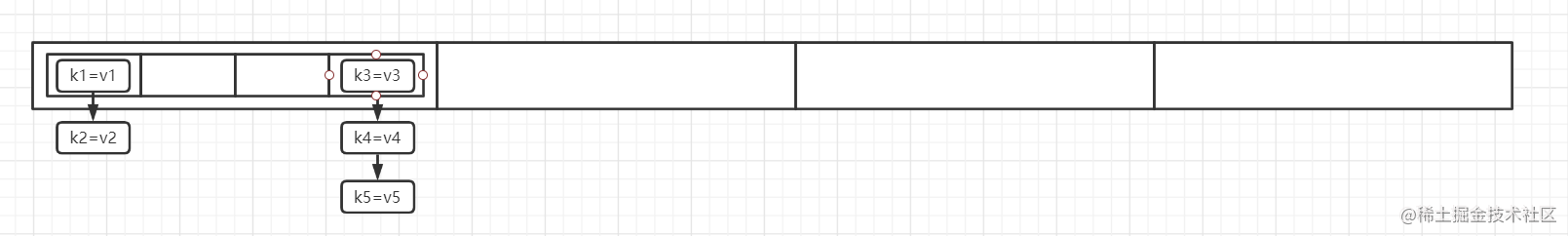JDK7中ConcurrentHashMap源码分析

一、Unsafe的使用

1.1、获取Unsafe

Unsafe, 顾名思义是不安全的, 其里面提供的方法都是较为底层的操作, 比如能够直接操作堆外内存, 比如
synchronized底层用到的monitorEnter和monitorExit同步功能等等, Unsafe对象默认的构造方法是private

Constructor<Unsafe> declaredConstructor = Unsafe.class.getDeclaredConstructor();
declaredConstructor.setAccessible( true );
Unsafe unsafe = declaredConstructor.newInstance();

1.2、Unsafe操作普通对象

class Person {

/* getter / setter */
}

Person person = new Person();
unsafe.putObjectVolatile( person,
unsafe.objectFieldOffset( Person.class.getDeclaredField( "username" ) ), "lisi" );

unsafe.objectFieldOffset方法用于获取一个对象的某个属性在内存中的偏移量, 通过上面的代码, 我们获取

unsafe还有一个putOrderedObject方法, 其参数和功能跟putObjectVolatile是一样的, 不同的地方在于, 前

1.3、Unsafe操作数组

String[] arr = new String[]{ "zhangsan1", "zhangsan2", "zhangsan3" };
long firstElementOffset = unsafe.arrayBaseOffset( arr.getClass() );
long perElementSize = unsafe.arrayIndexScale( arr.getClass() );
unsafe.putOrderedObject( arr, firstElementOffset + perElementSize , "lisi" );
System.out.println(Arrays.toString( arr ) );

二、ConcurrentHashMap源码分析

2.1、理论分析2.2、数据结构分析

static final class HashEntry<K,V> {
final int hash;
final K key;
volatile V value;
volatile HashEntry<K,V> next;
}

static final class Segment<K,V> extends ReentrantLock{
volatile HashEntry<K,V>[] table;
int modCount;
int count;
int threshold;
}

ReentrantLock保证了并发安全

2.3、构造方法分析

public ConcurrentHashMap() {
}

public ConcurrentHashMap(int initialCapacity, float loadFactor, int concurrencyLevel) {
............................
}

concurrencyLevel则是并发级别, 即用于表示Segment[]这个数组的长度, 然而因为有参构造方法是public

public ConcurrentHashMap(int initialCapacity, float loadFactor, int concurrencyLevel) {
if (concurrencyLevel > MAX_SEGMENTS)
concurrencyLevel = MAX_SEGMENTS;
// Find power-of-two sizes best matching arguments
int sshift = 0;
int ssize = 1;
while (ssize < concurrencyLevel) {
++sshift;
ssize <<= 1;
}
this.segmentShift = 32 - sshift;
..............................
}

Unsafe对象操作Segment数组中的元素的, 之后我们再进行分析

public ConcurrentHashMap(int initialCapacity, float loadFactor, int concurrencyLevel) {
if (initialCapacity > MAXIMUM_CAPACITY)
initialCapacity = MAXIMUM_CAPACITY;
int c = initialCapacity / ssize;
if (c * ssize < initialCapacity)
++c;

int cap = MIN_SEGMENT_TABLE_CAPACITY;
while (cap < c)
cap <<= 1;

.....................................
}

initialCapacity为17, 而ssize为16, 那么经过触发后得到的值就是1了, 所以此时会有余数, 这个时候会使

2的幂次方, 所以cap先设置为为最小的2的幂次方, 当其小于c的时候, 就进行左移操作, 从而获取到第一个大于

public ConcurrentHashMap(int initialCapacity, float loadFactor, int concurrencyLevel) {
// create segments and segments
Segment<K,V> s0 =
(HashEntry<K,V>[])new HashEntry[cap]);
Segment<K,V>[] ss = (Segment<K,V>[])new Segment[ssize];
UNSAFE.putOrderedObject(ss, SBASE, s0); // ordered write of segments
this.segments = ss;
}

2.4、put方法

1、整体分析

public V put(K key, V value) {
Segment<K,V> s;
if (value == null)
throw new NullPointerException();
int hash = hash(key);
int j = (hash >>> segmentShift) & segmentMask;
if ((s = (Segment<K,V>)UNSAFE.getObject          // nonvolatile; recheck
(segments, (j << SSHIFT) + SBASE)) == null) //  in ensureSegment
s = ensureSegment(j);
return s.put(key, hash, value, false);
}

(hash >>> segmentShift) & segmentMask, 这一段代码, 是用来计算key应该放在哪个Segment中的, 即计

(32 - 4 = 28)位, 从而只保留了高四位, 利用高四位与segmentMask进行与操作从而获取到一个合适的索引

Class sc = Segment[].class;
SBASE = UNSAFE.arrayBaseOffset(sc);
ss = UNSAFE.arrayIndexScale(sc);

(j << SSHIFT) + SBASE), 这段代码等价于j * UNSAFE.arrayIndexScale(Segment[].class) + c, 即获

if判断的功能就清晰了: 获取Segment[]数组索引位置为j的Segment对象

2、ensureSegment方法创建Segment对象

private Segment<K,V> ensureSegment(int k) {
final Segment<K,V>[] ss = this.segments;
long u = (k << SSHIFT) + SBASE; // raw offset
................
}

private Segment<K,V> ensureSegment(int k) {
..........................
Segment<K,V> seg;
if ((seg = (Segment<K,V>)UNSAFE.getObjectVolatile(ss, u)) == null) {
Segment<K,V> proto = ss; // use segment 0 as prototype
int cap = proto.table.length;
int threshold = (int)(cap * lf);
HashEntry<K,V>[] tab = (HashEntry<K,V>[])new HashEntry[cap];
if ((seg = (Segment<K,V>)UNSAFE.getObjectVolatile(ss, u))
== null) { // recheck
Segment<K,V> s = new Segment<K,V>(lf, threshold, tab);
while ((seg = (Segment<K,V>)UNSAFE.getObjectVolatile(ss, u))
== null) {
if (UNSAFE.compareAndSwapObject(ss, u, null, seg = s))
break;
}
}
}
return seg;
}

while循环加CAS就是用来保证多线程情况下并发安全的

3、Segment对象的put方法

final V put(K key, int hash, V value, boolean onlyIfAbsent) {
HashEntry<K,V> node = tryLock() ? null :
scanAndLockForPut(key, hash, value);
V oldValue;
try {
.....................
} finally {
unlock();
}
return oldValue;
}

put操作只会锁住一段数据, 即只会锁住一个Segment对象中的HashEntry[]数组, 而这个锁的来源是Segment

scanAndLockForPut方法不停的获取锁, 由于第一次的tryLock没有获取到锁, 此时为了能够做一些其他的事

final V put(K key, int hash, V value, boolean onlyIfAbsent) {
..........................................
HashEntry<K,V>[] tab = table;
int index = (tab.length - 1) & hash;
HashEntry<K,V> first = entryAt(tab, index);
for (HashEntry<K,V> e = first;;) {
if (e != null) {
K k;
if ((k = e.key) == key ||
(e.hash == hash && key.equals(k))) {
oldValue = e.value;
if (!onlyIfAbsent) {
e.value = value;
++modCount;
}
break;
}
e = e.next;
}
else {
if (node != null)
node.setNext(first);
else
node = new HashEntry<K,V>(hash, key, value, first);
int c = count + 1;
if (c > threshold && tab.length < MAXIMUM_CAPACITY)
rehash(node);
else
setEntryAt(tab, index, node);
++modCount;
count = c;
oldValue = null;
break;
}
}
..........................................
}

key, 进而创建的HashEntry对象, 这个时候, 就直接将其next指向该Segment中HashEntry对应索引下的第一个

rehash方法相对有点复杂, 主要是ConcurrentHashMap的作者想要提高性能才使得这么复杂的, 简单的实现就

A -> B -> C -> D -> E, 如果C、D、E这三个元素计算出在新的数组中的索引位置是相同的(比如新的索引位

newTable[Y] = C

2.5、get方法

get方法就不进行仔细分析了, 有了put方法的基础, get方法看起来会非常的简单, 这里需要提及的一点是,